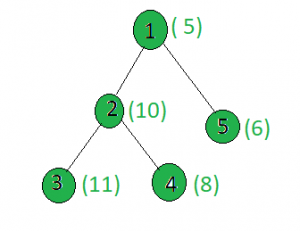# Find the node whose sum with X has minimum set bits

Given a tree, and the weights of all the nodes and an integer x, the task is to find a node i such that weight[i] + x gives the minimum setbits, If two or more nodes have the same count of set bits when added with x then find the one with the minimum value.

Examples:

Input:x = 15
Output: 1
Node 1: setbits(5 + 15) = 2
Node 2: setbits(10 + 15) = 3
Node 3: setbits(11 + 15) = 3
Node 4: setbits(8 + 15) = 4
Node 5: setbits(6 + 15) = 3

## Recommended: Please try your approach on {IDE} first, before moving on to the solution.

Approach: Perform dfs on the tree and keep track of the node whose sum with x has minimum set bits. If two or more nodes have equal count of set bits then choose the one with the minimum number.

Below is the implementation of the above approach:

## C++

 `// C++ implementation of the approach ` `#include ` `using` `namespace` `std; ` ` `  `int` `minimum = INT_MAX, x, ans = INT_MAX; ` ` `  `vector<``int``> graph; ` `vector<``int``> weight(100); ` ` `  `// Function to perform dfs to find ` `// the minimum set bits value ` `void` `dfs(``int` `node, ``int` `parent) ` `{ ` `    ``// If current set bits value is smaller than ` `    ``// the current minimum ` `    ``int` `a = __builtin_popcount(weight[node] + x); ` `    ``if` `(minimum > a) { ` `        ``minimum = a; ` `        ``ans = node; ` `    ``} ` ` `  `    ``// If count is equal to the minimum ` `    ``// then choose the node with minimum value ` `    ``else` `if` `(minimum == a) ` `        ``ans = min(ans, node); ` ` `  `    ``for` `(``int` `to : graph[node]) { ` `        ``if` `(to == parent) ` `            ``continue``; ` `        ``dfs(to, node); ` `    ``} ` `} ` ` `  `// Driver code ` `int` `main() ` `{ ` `    ``x = 15; ` ` `  `    ``// Weights of the node ` `    ``weight = 5; ` `    ``weight = 10; ` `    ``weight = 11; ` `    ``weight = 8; ` `    ``weight = 6; ` ` `  `    ``// Edges of the tree ` `    ``graph.push_back(2); ` `    ``graph.push_back(3); ` `    ``graph.push_back(4); ` `    ``graph.push_back(5); ` ` `  `    ``dfs(1, 1); ` ` `  `    ``cout << ans; ` ` `  `    ``return` `0; ` `} `

## Python3

 `# Python3 implementation of the approach ` `from` `sys ``import` `maxsize ` ` `  `minimum, x, ans ``=` `maxsize, ``None``, maxsize ` ` `  `graph ``=` `[[] ``for` `i ``in` `range``(``100``)] ` `weight ``=` `[``0``] ``*` `100` ` `  `# Function to perform dfs to find ` `# the minimum set bits value ` `def` `dfs(node, parent): ` `    ``global` `x, ans, graph, weight, minimum ` ` `  `    ``# If current set bits value is greater than ` `    ``# the current minimum ` `    ``a ``=` `bin``(weight[node] ``+` `x).count(``'1'``) ` ` `  `    ``if` `minimum > a: ` `        ``minimum ``=` `a ` `        ``ans ``=` `node ` ` `  `    ``# If count is equal to the minimum ` `    ``# then choose the node with minimum value ` `    ``elif` `minimum ``=``=` `a: ` `        ``ans ``=` `min``(ans, node) ` ` `  `    ``for` `to ``in` `graph[node]: ` `        ``if` `to ``=``=` `parent: ` `            ``continue` `        ``dfs(to, node) ` ` `  `# Driver Code ` `if` `__name__ ``=``=` `"__main__"``: ` ` `  `    ``x ``=` `15` ` `  `    ``# Weights of the node ` `    ``weight[``1``] ``=` `5` `    ``weight[``2``] ``=` `10` `    ``weight[``3``] ``=` `11` `    ``weight[``4``] ``=` `8` `    ``weight[``5``] ``=` `6` ` `  `    ``# Edges of the tree ` `    ``graph[``1``].append(``2``) ` `    ``graph[``2``].append(``3``) ` `    ``graph[``2``].append(``4``) ` `    ``graph[``1``].append(``5``) ` ` `  `    ``dfs(``1``, ``1``) ` ` `  `    ``print``(ans) ` ` `  `# This code is contributed by ` `# sanjeev2552 `

Output:

```1
```

Complexity Analysis:

• Time Complexity : O(N).
In dfs, every node of the tree is processed once and hence the complexity due to the dfs is O(N) if there are total N nodes in the tree. Also, for processing each node the builtin_popcount() function is used which has a complexity of O(c) where c is a constant and since this complexity is constant, it doesnot affect the overall time comlexity. Therefore, the time complexity is O(N).
• Auxiliary Space : O(1).
Any extra space is not required, so the space complexity is constant.

Don’t stop now and take your learning to the next level. Learn all the important concepts of Data Structures and Algorithms with the help of the most trusted course: DSA Self Paced. Become industry ready at a student-friendly price.

My Personal Notes arrow_drop_upCheck out this Author's contributed articles.

If you like GeeksforGeeks and would like to contribute, you can also write an article using contribute.geeksforgeeks.org or mail your article to contribute@geeksforgeeks.org. See your article appearing on the GeeksforGeeks main page and help other Geeks.

Please Improve this article if you find anything incorrect by clicking on the "Improve Article" button below.

Improved By : sanjeev2552, muskan_garg

Article Tags :

Be the First to upvote.

Please write to us at contribute@geeksforgeeks.org to report any issue with the above content.